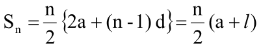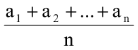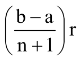# Arithmetic Progressions (A.P.)

## Arithmetic Progressions (A.P.)

(a) If a is the first term and d is the common difference then AP can be written as

a, a + d, a + 2d, . . . a + (n-1) d.

with term Tn = a + (n-1) d = l (last term)

where d = Tn – Tn-1

with term from the last Tn = l – (n - 1) d

(b) Sum of first n termand Tn = Sn – Sn-1

(c) Arithmetic mean of any n positive numbers a1 a2 . . . an is

AM =(d) If n arithmetic means A1 A2 . . . An are inserted between a and b then

Ar = a +1 ≤ r ≤ n and A0 = a, An+1 = b.